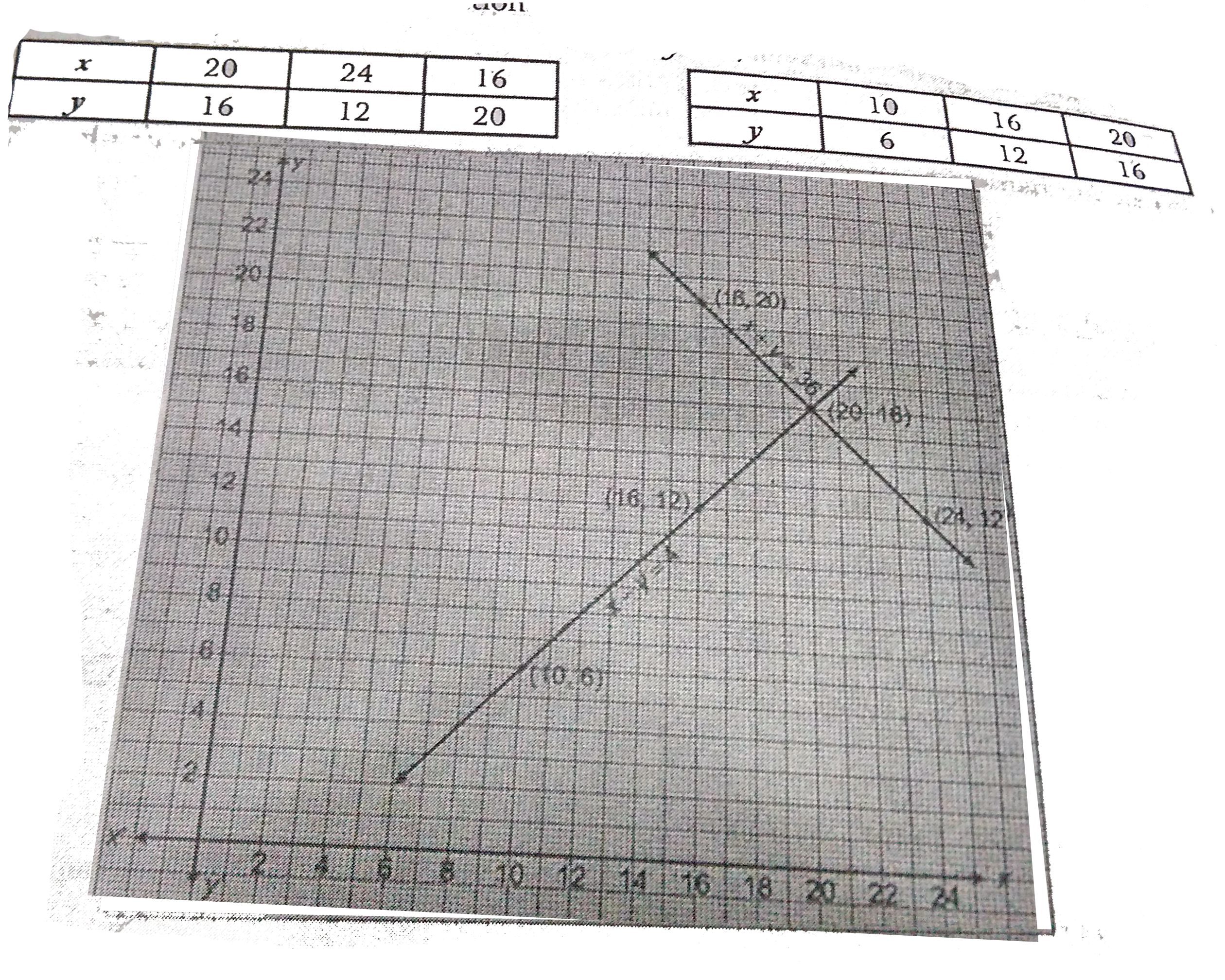# Half the perimeter of a rectangular garden, whose length is 4 m more than its width, is 36 m. Find the dimensions of the garden by graphical method.

122 views

closed
Half the perimeter of a rectangular garden, whose length is 4 m more than its width, is 36 m. Find the dimensions of the garden by graphical method.

by (43.8k points)
selected by

Let the length of the garden be x metre and its width be y metre.
Then, perimeter of rectangular garden = 2 (length + width) - 2 (x + y)
Therefore, half perimeter = x + y
But it is given that half perimeter = 36 m
therefore x + y = 36 ...(1)
Also, x = y + 4, i.e., x - y = 4 ...(2)
For finding the solution of equation (1) and (2) graphically, we form the following table :Draw the graphs by joining points (20, 16), (16, 20) and (24, 12), and points (10, 6), (16, 12) and (20, 16). The two lines intersect at point (20, 16) as shown in the graph. Hence, length = 20 m and width = 16m